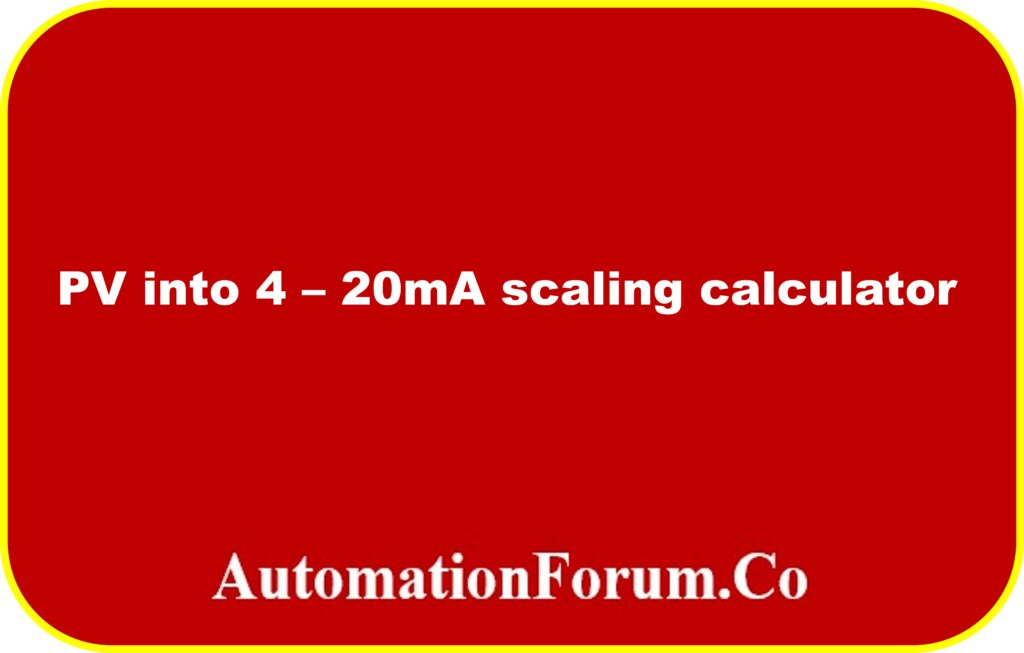# PV to 4-20mA scaling calculator4-20 mA signals are used to transmit physical process values, such as temperature, pressure, liquid level, or other physical quantities. The current in the loop represents the equivalent physical process value.

## Example:

A temperature transmitter with a temperature range of 0 to 1000 degrees Celsius produces 7.2 mA in an analogue loop, which is equal to the degree temperature being measured at 200 degrees.

The following “PV into 4-20mA scaling calculator” transforms the measured value to the current:

The following calculations provide the basis for the PV into 4-20mA scaling calculator:

Formula for changing a physical value (Pv) to a current (I):

I=[(I high – I low)/(Pv high – Pv low)]*(Pv-Pv low)+I low

In this article, scaling is used to establish the relationship between a field value (in this case, mA) and a process value (e.g. Temperature, Pressure, Level).

The electrical current loop signal is converted into a physical value using a linear characteristic. This feature is used to describe the relationship between the current signal and physical value.

It is important to take extra precautions when converting from a physical value with a starting value other than zero. a pressure indication with a range of -1 to 1 bar, for example. 12 mA of current are flowing at a pressure of 0 bar. In this situation, it is advised that you utilise a scaling calculator because the 4 mA starting current makes the relationship between the physical value and current irrational.

It could be necessary to test instruments using a  simulator when working with real – life conditions. Because the simulator allows manual control of the process value in the loop, scaling can be evaluated and checked relatively easily.